## Excel Formula: Add minutes to time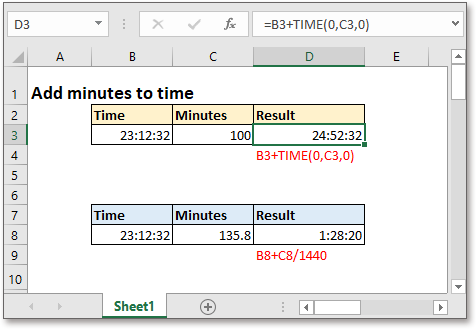This tutorial provides formulas and explains how to add minutes to time in Excel.

### Add whole minutes to time

Generic formula:

 Start_time+TIME(0,minutes,0)

Arguments

 Start_time: the time you want to add minutes to. Hours: the number of minutes you want to add to time. It must be a positive whole number. If it is negative, the formula returns an error value #NUM!, if it is decimal number, such as 30.1, it reckons 30.1 as 30.

Return value

Return a value in time format.

How this formula work

For example, there is a time data in cell B3, and the number of minutes you want to add is displayed in cell C3, please use below formula:

 =B3+TIME(0,C3,0)

Or

 =B3+TIME(0,36,0)

Press Enter key then the result is shown.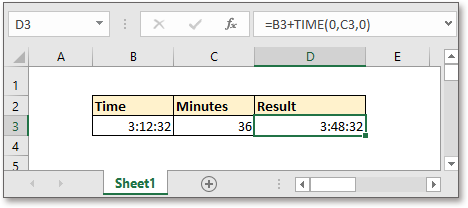Explanation

Generally, TIME function is used to convert decimal numbers to a time in format hh:mm:ss. Here in this formula, it changes the number of minutes (36) to a time (00:36:00), and then the entire formula =B3+TIME(0,C3,0) is calculated to: 3:12:32+00:36:00.

Remark

If you want to subtract the minutes from time, please use below formula

 MOD(Start_time-TIME(0,minutes,0),1)

For example, there is a time in cell B12, and the number of minutes you want to subtract is displayed in cell C13, please use below formula:

=MOD(B12-TIME(0,20,0),1)

Or

=MOD(B13-TIME(0,C12,0),1)

Press Enter key then the result is shown.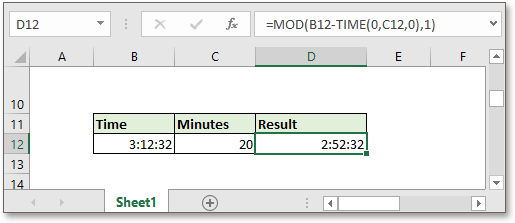Explanation

In general, it does not allow the negative time in Excel, using the MOD function to flip the negative values to positive will get a correct time.

### Add decimal minutes to time

Generic formula:

 Start_time+minutes/1440

Arguments

 Start_time: the time you want to add minutes to. Minutes: the decimal minutes you want to add to time.

Return value

Return a value in time format.

How this formula work

For example, the start time value is in cell B8, the decimal minutes you want to add is displayed in cell C8, please use below formula:

 =B8+C8/1440

Or

 =B8+135.8/1440

Press Enter key then the result is shown.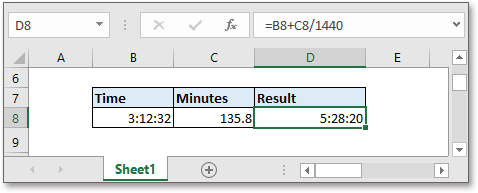Explanation

There are 1440 minutes in a day, and one minute is 1/1440 day.

Remark

If you want to subtract the minutes from time, please use below formula

 MOD(Start_time-minutes/1440,1)

For example, there is a time in cell B18, and the number of minutes you want to subtract is displayed in cell C18, please use below formula:

==MOD(B18-C18/1440,1)

Or

=MOD(B18-200/1440,1)

Press Enter key then the result is shown.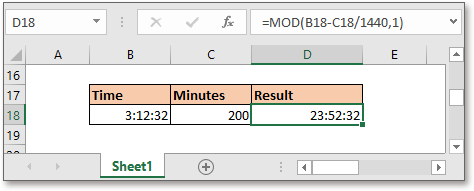Explanation

Generally speaking, it does not allow the negative time in Excel, using the MOD function to flip the negative values to positive will get a correct time.

### Note:

1. The number of hours can be a decimal number while using the formula
Start_time+minutes/1440

2. If the result is over 24 hours, just format the result as [h]:mm:ss format in the Custom section under Number tab of the Format Cells dialog.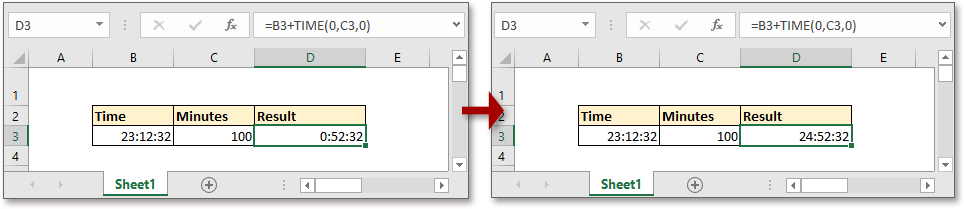### Sample File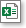Click to download sample file

#### Relative Formulas

• Add Hours Minutes Seconds To Time
Supposing there is a list of time values in Excel, now you want to add 3 hours 45 minutes and 20 seconds to the time values, Excel provides two formulas to help you.
In this tutorial, it introduces the formulas to add whole hours or decimal hours to a given time in Excel.
• Get Week Number From Date In Excel
In Excel, how could you get week number from the given date quickly and easily? Normally, the WEEKNUM function can help you to solve this task as quickly as possible.
• Get Work Hours Between Two Dates In Excel
The NETWORKDAYS function in Excel can help you to get the net workdays between two dates, and then multiply the number of working hours per workday to get the total work hours.

#### Relative Functions

• Excel Date Function
DATE function can help us to combine year, month and day numbers from separate cells to a valid date.
• Excel WEEKDAY Function
WEEKDAY function returns an integer number from 1 to 7 to represent the day of the week for a given date in Excel.
• Excel WORKDAY Function
The WORKDAY is used to add a specified number of workdays to a started date and returns the future or past date in a serial number format.
• Excel WORKDAY.INTL Function
WORKDAY.INTL function adds a specified number of workdays to a start date and returns a workday in the future or past.
• Excel YEAR Function
The YEAR function returns the year based on the given date in a 4-digit serial number format.

### .kte-bottom-general { max-width: 1220px; margin: 0 auto; font-family: arial; /* border: solid 1px #f1f0ef; */ } .kte-bottom-general img { display: block; margin: 0 auto; } .tex-content { margin-right: 10px; } The Best Office Productivity Tools Kutools for Excel - Helps You To Stand Out From Crowd Popular Features: Find, Highlight or Identify Duplicates  |  Delete Blank Rows  |  Combine Columns or Cells without Losing Data  |  Round without Formula ... Super VLookup: Multiple Criteria  |  Multiple Value  |  Across Multi-Sheets  |  Fuzzy Lookup... Adv. Drop-down List: Easy Drop Down List  |  Dependent Drop Down List  |  Multi-select Drop Down List... Column Manager: Add a Specific Number of Columns  |  Move Columns  |  Toggle Visibility Status of Hidden Columns  |  Compare Columns to Select Same & Different Cells ... Featured Features: Grid Focus  |  Design View  |  Big Formula Bar  |  Workbook & Sheet Manager | Resource Library (Auto Text)  |  Date Picker  |  Combine Worksheets  |  Encrypt/Decrypt Cells  |  Send Emails by List  |  Super Filter  |  Special Filter (filter bold/italic/strikethrough...) ... Top 15 Toolsets:  12 Text Tools (Add Text, Remove Characters ...)  |  50+ Chart Types (Gantt Chart ...)  |  40+ Practical Formulas (Calculate age based on birthday ...)  |  19 Insertion Tools (Insert QR Code, Insert Picture from Path ...)  |  12 Conversion Tools (Numbers to Words, Currency Conversion ...)  |  7 Merge & Split Tools (Advanced Combine Rows, Split Excel Cells ...)  |  ... and more Kutools for Excel Boasts Over 300 Features, Ensuring That What You Need is Just A Click Away...Read MoreFree Download Office Tab - Enable Tabbed Reading and Editing in Microsoft Office (include Excel) One second to switch between dozens of open documents! Reduce hundreds of mouse clicks for you every day, say goodbye to mouse hand. Increases your productivity by 50% when viewing and editing multiple documents. Brings Efficient Tabs to Office (include Excel), Just Like Chrome, Edge and Firefox.Read More Free Download

No ratings yet. Be the first to rate!
This comment was minimized by the moderator on the site
Hello and thank you for all the info provided.

With all due respect, someone needs to proofread your page, for there are several inconsistencies such as:

(right off the bat...)
Start_time+TIME(0,minutes,0)
Arguments
Start_time: ...
Hours: the number of...

Hours? Shouldn't it be TIME?

For example, there is a time in cell B12, and the number of minutes you want to subtract is displayed in cell C13, please use below formula:
=MOD(B12-TIME(0,20,0),1)
Or
=MOD(B13-TIME(0,C12,0),1)

Why did B12 become B13?
Where is C13 used?
These are two discrepancies in just one example.

Generally speaking, it does not allow the negative time in Excel
it???

There are 1440 minutes in a day, and one minute is 1/1440 day.
There are 1440 minutes in a day, so... or therefore,...

It seems you are hiring illegals who do not speak English all that well to write your material.
There are no comments posted here yet